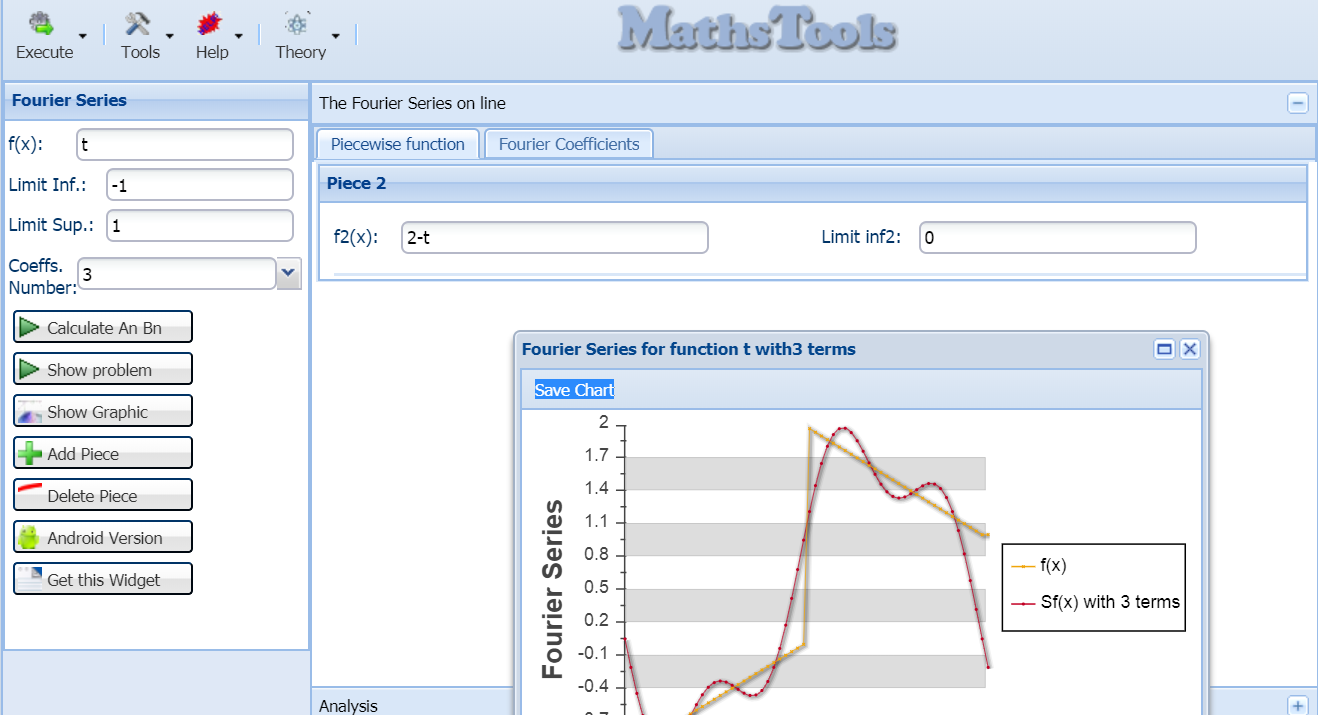# Fourier Series Calculator

Fourier Series Calculator is an online application on the Fourier series to calculate the Fourier coefficients of one real variable functions. Also can be done the graphical representation of the function and its Fourier series with the number of coefficients desired

### Inputs

Fourier Series Calculator allows you to enter picewise-functions defined up to 5 pieces, enter the following

0) Select the number of coefficients to calculate, in the combo box labeled "Select Coefs.Number".
1) Enter the lower integration limit (full range) in the field labeled "Limit Inf.".
2) Enter the upper integration limit (the total range) in the field labeled "Limit Sup.".
3) Enter the function of the variable x. Some examples are
if f(x) = e2x -> enter e^(2*x)
if f(x,y) = sin e2x -> enter sin(e^(2*x))
4) If the function is defined piecewise, enter the upper limit of the first interval in the field labeled "Sub-interval 1" and enter the function from that point to the next interval if more or "Limit inf." value entered in step 2)
5)If there are more pieces enter the upper end of the next subinterval in the field labeled "Sub-interval 2", and enter the function from that point to the next interval if more or "Limit inf." value entered in step 2)
6)If more pieces repeat the previous step in the following fields, Fourier Series Calculator lets you enter up to 4 subintervals.

### Fourier Series Calculator Outputs

To calculate the coefficients of the Fourier series click "Fourier Series": After a few seconds, a window opens showing the An and An Fourier series coefficients for the function introduced, also will show some statistics of the calculations.

Click on "Show Graph" if you want to see the graphic representation of the function and the previously calculated series

Push in "df(x)/dx" To obtain the analytical derivative of the function

Push inn "Integral[a, b]" To obtain the primitive of the function in the interval introduced

Fourier Series Calculator has, in the precision limitation in calculations up to 16 decimal digits. Note that the precision in the calculation of each coefficient depends on size of interval entered, for an interval of length 2π the error is roughly o(10-7).
At same time, the maximum processing time is 20 seconds, after that time if no solution is found, Fourier Series Calculator will stop the execution, for higher execution times please use the applet on this website.

Fourier Series Calculator does not require installation of any kind, just a browser with javascript support.

# Was useful? want add anything?

Post here

### Carlos:

2020-06-05 07:24:30
Hi John.

Sorry. Discrete pieces are not supported here.

### john:

2020-06-05 07:24:30
how to get input

f(x)= 1 (when -1<=x<0),
1/2 (when x=0) ,
x (when 0

### Carlos:

2020-05-06 18:44:12
Hi Sam.

I don't understadn fist piece of your function i will assume that you're trying:

y(t)=t for -1 y(t)=2-t for 0
In such case you can procced as :Note that you can use t as independent variable, instead x.

### Sam:

2020-05-04 18:44:12
fourier series of

y=(t) for 0 y(t)=2-t for 0
i don't understand how to put in calculator hoping someone can for me

I need all parts a0 an bn and the final

2019-02-11 00:41:14
Hi, Sam

Thanks for your comment. You can input data as this screenshot:Regards.

### sam:

2019-08-21 04:16:46

If the

Fourier Series for the function f(x) , defined on (2,2), where

f(x)={ -1 , -2<x<0
{ 2 , 0<x<2

how do we process the above?
Thank you

2016-08-04 14:36:39
Hi Lawrence!.

Thanks for your comment. In fact, the a0 coefficient appears divided by 2 in calculator results.
For example, you can calculate the coefficients for function:

f(x) = -x ,if -pi<x<0
f(x) = x ,if 0<x<pi
(Please ignore the great or equaly signs and consider all interval [-pi, pi] covered).

At this case coefficient a0=PI, but application says that a0 value is PI/2.

### Laurence Le Vay:

2016-08-03 22:31:39
Hi There, I am studying my Mechanical Engineering degree, and studying Fourier Series.
I am constantly getting different values for a0 compared from working out the formula: a0 = 1/Pi Integral Between 2Pi and 0 for the function.
For instance I am looking at the wave:

f(t) = t , if 0<t<Pi
f(t) = 2Pi-t , if Pi<t<2Pi

My workings out show a0 should be 2*Pi.

Your calculator shows a0 is 0.5*Pi I agree with the values for an and bn, and the graphic looks correct.
I just don't understand the discrepency for a0!

Hope you can help!

Many thanks
Laurence :)

PS Great calcuator, thank you for your work

2012-11-18 23:13:40
Hi, Jhon
OK, this change would be useful. Please give us some days to make it.

### John:

2012-11-18 17:17:42
Very useful, thanks. Would be nice if it was possible to view intercepts and y values at certain values at specific values of x.

Post here